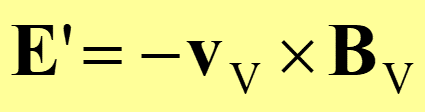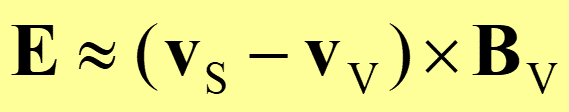# Modified Josephson relation

 It is well known and widely accepted, that in superconductors effective electric field (for stationary state defined as gradient of the electrochemical potential) is proportional to the velocity of the vortex lattice. We proposed that the true electric field (for stationary state defined as gradient of the scalar potential) is given by so called modified Josephson relation stating that it is proportional to the relative velocity of the vortex lattice in respect to the velocity of the superconducting fluid. see the paper: Modified Josephson relation J. Koláček, P.Lipavský International Journal of Modern Physics B 17, 3407 (2003) Effective electric field in type II superconductor is given by Josephson relationThe true electric field in the bulk type II superconductor at low temperatures is approximated by modified Josephson relation